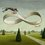# $e$ and $\pi$

Theoretically, can $\pi$ contain the entire sequence of digits of $e$ ? Also, can $e$ contain the entire sequence of digits of $\pi$?Note by Hobart Pao
5 years, 5 months ago

This discussion board is a place to discuss our Daily Challenges and the math and science related to those challenges. Explanations are more than just a solution — they should explain the steps and thinking strategies that you used to obtain the solution. Comments should further the discussion of math and science.

When posting on Brilliant:

• Use the emojis to react to an explanation, whether you're congratulating a job well done , or just really confused .
• Ask specific questions about the challenge or the steps in somebody's explanation. Well-posed questions can add a lot to the discussion, but posting "I don't understand!" doesn't help anyone.
• Try to contribute something new to the discussion, whether it is an extension, generalization or other idea related to the challenge.

MarkdownAppears as
*italics* or _italics_ italics
**bold** or __bold__ bold
- bulleted- list
• bulleted
• list
1. numbered2. list
1. numbered
2. list
Note: you must add a full line of space before and after lists for them to show up correctly
paragraph 1paragraph 2

paragraph 1

paragraph 2

[example link](https://brilliant.org)example link
> This is a quote
This is a quote
    # I indented these lines
# 4 spaces, and now they show
# up as a code block.

print "hello world"
# I indented these lines
# 4 spaces, and now they show
# up as a code block.

print "hello world"
MathAppears as
Remember to wrap math in $$ ... $$ or $ ... $ to ensure proper formatting.
2 \times 3 $2 \times 3$
2^{34} $2^{34}$
a_{i-1} $a_{i-1}$
\frac{2}{3} $\frac{2}{3}$
\sqrt{2} $\sqrt{2}$
\sum_{i=1}^3 $\sum_{i=1}^3$
\sin \theta $\sin \theta$
\boxed{123} $\boxed{123}$

Sort by:

This reminds me of Banach–Tarski paradox.

Reshared!

- 5 years, 5 months ago

Not necesary, I think, is like saying that the secuence of digits of $e$ is a subset of the secuence of digits of $\pi$

- 5 years, 5 months ago

I mean I've seen these articles where they say "Any/all of your numerical passwords, everyone's birthday, blah blah blah are contained within pi" or something like that. This may be an obvious question, but where's the limit on length of a sequence of digits that can fit into pi?

- 5 years, 5 months ago

Yes! I'll give a constructive argument in a few minutes

- 5 years, 5 months ago

...

• Digits of pi in e = 2.7182818284590452353602874713526624977572470936999595749669676277240766303535475945713821785251664274274663919320030599218174136

• Digits of e in pi = 3.1415926535897932384626433832795028841971693993751058209749445923078164062862089986280348253421170679821480865132823066470938446095505822317253594081056239

- 5 years, 5 months ago

I meant seeing 314159265358979323846...somewhere in $e$ eventually. Without skipping digits. Because the way you did it, of course you could fit all the digits eventually lol

- 5 years, 5 months ago

If it did, then the following would hold:

$e = x + 10^{-i}\pi$

where x is a rational number and i is an integer. I do not think this is true. Although, I cannot prove it.

- 5 years, 5 months ago

ah! interesting...why does x have to be rational? It could be the sum of two irrationals, right? And since $\pi$ has infinite digits, will your rational number have decimals? Because you have to then avoid modifying the $\pi$ portion and maybe have a.0000000000000000000000000000000000000000000000...11111111111111111111111111111111111111111111111111...0000000000000000000000000 just as an example. I could be wrong because I haven't slept much...lol

- 5 years, 5 months ago

Let's say that e was 2.72831415...

In this case, x= 2.728 is the rational part

- 5 years, 5 months ago

so you're talking about putting $\pi$ at the end of $e$? That doesn't make much sense

- 5 years, 5 months ago

because it could just be somewhere in the "middle" of $e$ because $e$ doesn't end

- 5 years, 5 months ago

$\pi$ is irrational and hence doesn't have a terminating decimal expansion; how can it be in the middle of $e$?

- 5 years, 5 months ago

but nonetheless, that is interesting! Would it be okay if $\pi$ were at the end of $e$? Because both are irrational. Would it matter?

- 5 years, 5 months ago

A note: Only one of them can be true. I shall prove it, by Contradiction.

Let $e$ have $a$ digits and $\pi$ have $b$ digits.

Then, $a \leq b \leq a$.

Since a=a, a=b.

a = b implies that $\pi = e$.

- 5 years, 5 months ago

No, both numbers are irrational and they don't terminate, so you can't say that $e$ have $a$ digits and $\pi$ have $b$ digits.

- 5 years, 4 months ago